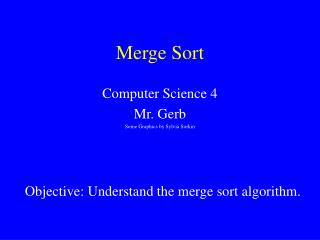DownloadDownload PresentationMerge Sort

# Merge Sort

Download Presentation## Merge Sort

- - - - - - - - - - - - - - - - - - - - - - - - - - - E N D - - - - - - - - - - - - - - - - - - - - - - - - - - -
##### Presentation Transcript

1. Merge Sort Computer Science 4 Mr. Gerb Some Graphics by Sylvia Sorkin Reference: Objective: Understand the merge sort algorithm.

2. Divide and Conquer Sorts • Selection sort and bubble sort are both O(N2) sorts. • Both are in a family of sorts that tend to compare each item with each other item. • O(N2) sorts are thought of as very slow sorts. • “Divide and conquer” sorts • Tend to divide the list up as evenly as possible • Sort each half • Combine the two. • The next two sorts we study will be in this category • These sorts tend to require O(N*LogN) comparisons.

3. Merge Sort Algorithm Cut the array in half. Sort the left half. Sort the right half. Merge the two sorted halves into one sorted array. 74 36 . . . 95 75 29 . . . 52 [first] [middle] [middle + 1] [last] 29 52 . . . 75 36 74 . . . 95

4. Merging Two Sorted Arrays Consider the first item in each array 9 22 61 88 9 14 23 31 70

5. Merging Two Sorted Arrays 9 22 61 88 9 14 14 23 31 70

6. Merging Two Sorted Arrays 9 22 61 88 9 14 22 14 23 31 70

7. Merging Two Sorted Arrays 9 22 61 88 9 14 22 23 14 23 31 70

8. Merging Two Sorted Arrays 9 22 61 88 9 14 22 23 31 14 23 31 70

9. Merging Two Sorted Arrays 9 22 61 88 9 14 22 23 31 61 14 23 31 70

10. Merging Two Sorted Arrays 9 22 61 88 9 14 22 23 31 61 70 14 23 31 70

11. Merging Two Sorted Arrays 9 22 61 88 9 14 22 23 31 88 61 70 14 23 31 70

12. Analysis of Merge Sort • Each time the list is split, most items need to be compared with another. • Therefore for each split, O(N) comparisons are needed. • How many times can you split a list of N elements in half? • For N=2, 1, for N=4, 2, for N=8, 3, etc. • In general LogN • Therefore merge sort requires O(N*LogN) comparisons. • This holds true both for worst case and average case.

13. Disadvantages of Merge Sort • We’ve seen that merge sort has a very good average and worst case. • What’s wrong with it? • Need a place to merge from • Therefore requires a block of member O(N) in size. • This is not a problem with bubble sort and selection sort, that do their job with swapping. • Also not a problem if you use merge sort to sort items in a linked list. Merge sort is well suited for linked lists.

14. Summary • O(N2) sorts are slow • Merge sort is faster • Splits list in half • Sorts each recursively • Merges the two together • Merge sort runs in O(N*LogN) time. • Requires substantial extra memory • Well suited to sort linked lists.